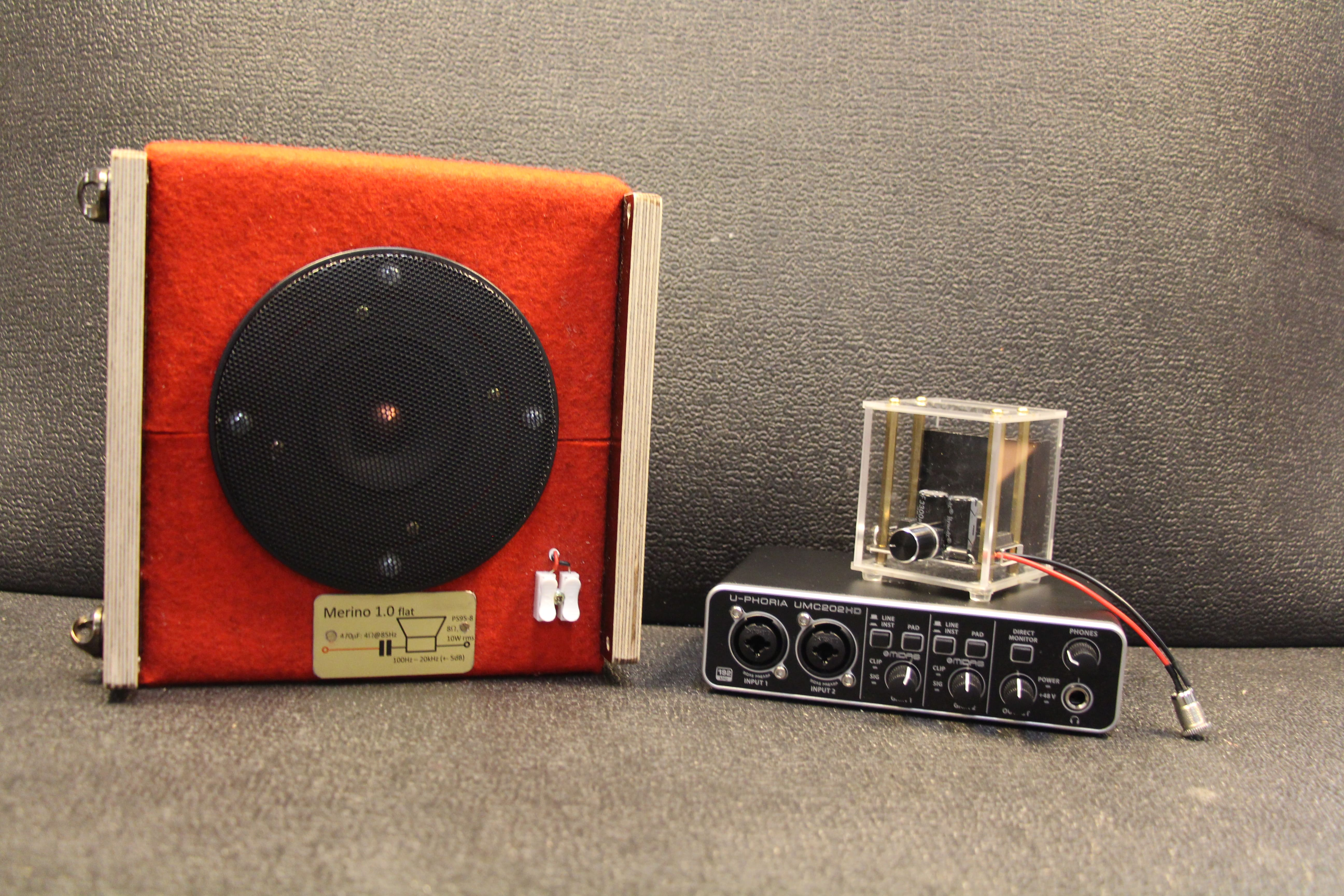## The ICIR Corpus - DatabaseContents:

We supply a data base with all data stored with 48 kHz. We provide MATLAB programs to convert the sample rate to the need of the user (8, 12, 16, 22.5, 24, 32, 44.1 kHz) and we provide programs to estimate impulse responses with the ‘user’ sample rate.

A data base could contain the impulse responses and/or the original data the impulse responses have been calculated from (measurement noise data). Our idea is that the data base should only contain the original data and the user ‘always’ calculates the impulses responses he/she needs. If the user has a specific sampling rate < 48 kHz, he may resample the original data once and store in a separate directory.

The advantage storing the original noise data only is that the most possible information for analysis and impulse response calculations with different methods is available. The disadvantage is that the original measurement noise data need much more storage space (48 kHz of e.g. 20 sec length) than the finally estimated impulse responses would need (e.g. 32 kHz of 0.1 sec length).

We supply only one method to estimate the impulse responses from measurement noise data, based on the power spectral densities of the measurement noise data. This method is implemented in the MATLAB function tfestimate. The length of the involved FFT needs to be (much) longer than the true impulse response. If we assume a max. reverberation time T60 of about 100 msec (with 48 kHz), we need 48.000 * 0.1= 4.800 samples, but should use for the estimation at least about 10.000 samples. Longer reverberation ( impulse responses require higher values. After the estimation the user may decide to use a shorter length of the estimate (cut the estimated impulse response, e.g. N = 8192 gives a length of h of 8192, h_short length = 2048).

For recording the measurement noise data we assume the user uses either 48 or 96kHz sampling frequency. We recommend 48 kHz. If the user has used 96 kHz for recording, a first downsampling to 48 kHz should we performed. The data base should contain data sampled with 48 kHz only.

#### Data Base structure

Sampling rate and file format is fixed to 48 kHz and .wav format. All this data are in a directory called data48. In summary we have:

file content
data 48 all noise recording: measurement noise, road noise, speech (write protected)
user 48, user44, user... resampled ‘noise’ from data 48 and estimated impulse responses
MAT MATLAB support programs
Docu documentation and data sheets
raw96 work directory in case recording was done with 96kHz, optional
raw48 work directory for standard recordings, 48kHz, optional
test work directory for testing